### Home > MC2 > Chapter 9 > Lesson 9.3.1 > Problem9-81

9-81.

Find the missing side lengths of each rectangle (a and c) or square (b and d). Homework Help ✎

 a. b. c. d.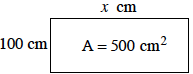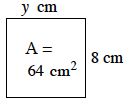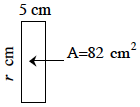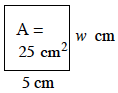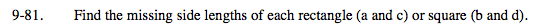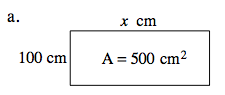To find the area of a rectangle, multiply the length
by the width.

100 cm ( x cm ) = 500 cm2

Solve for x.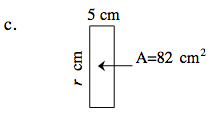See (a).

16.4 cm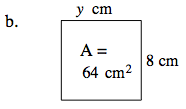Recall the properties of a square. All the side lengths must be equal.

Since one side is already given, we can conclude that the other side is also 8 cm.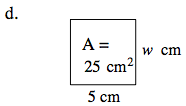See (b).